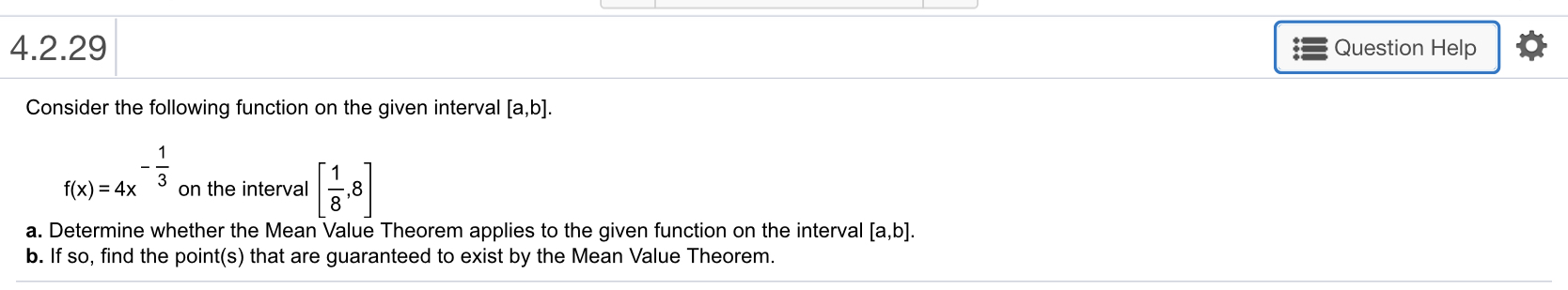# 4.2.29 Question Help Consider the following function on the given interval [a,b] f(x)-4x on the interval ,8 a. Determine whether the Mean Value Theorem applies to the given function on the interval [a,b] b. If so, find the point(s) that are guaranteed to exist by the Mean Value Theorem

Questionhelp_outlineImage Transcriptionclose4.2.29 Question Help Consider the following function on the given interval [a,b] f(x)-4x on the interval ,8 a. Determine whether the Mean Value Theorem applies to the given function on the interval [a,b] b. If so, find the point(s) that are guaranteed to exist by the Mean Value Theorem fullscreen

### Want to see this answer and more?

Experts are waiting 24/7 to provide step-by-step solutions in as fast as 30 minutes!*

*Response times vary by subject and question complexity. Median response time is 34 minutes and may be longer for new subjects.
Tagged in
MathCalculus

### Derivative